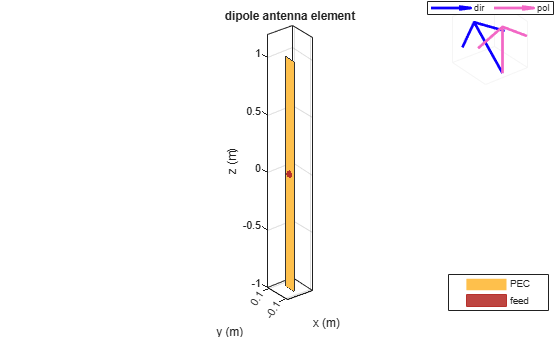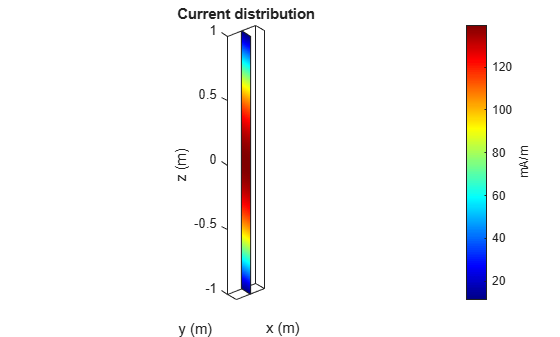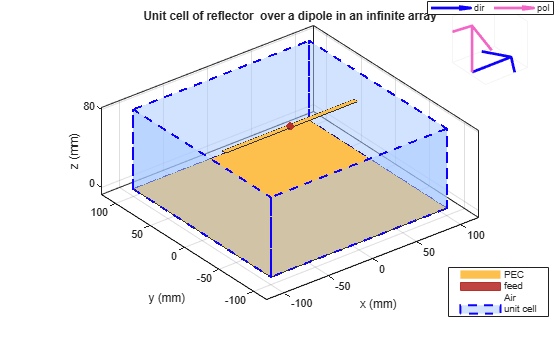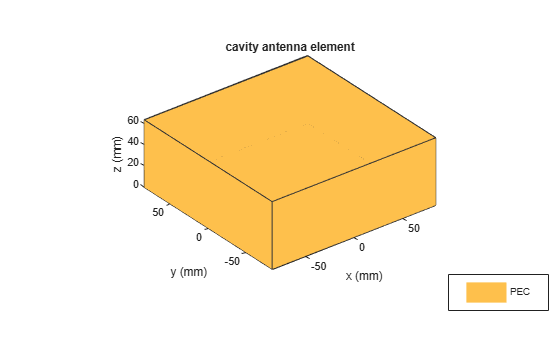# planeWaveExcitation

Create plane wave excitation environment for antenna or array

## Description

The `planeWaveExcitation` object creates an environment in which a plane wave excites an antenna or array. Plane wave excitation is a scattering solution that solves the receiver antenna problem.

## Creation

### Syntax

``h = planeWaveExcitation``
``h = planeWaveExcitation(Name=Value)``

### Description

example

````h = planeWaveExcitation` creates an environment in which a plane wave excites an antenna or array. The default receiver antenna is a dipole that is excited by a plane wave travelling along the positive x-axis with a z-polarization.```

example

````h = planeWaveExcitation(Name=Value)` sets Properties using one or more name-value arguments. `Name` is the property name and `Value` is the corresponding value. You can specify several name-value arguments in any order as `Name1=Value1`, `...`, `NameN=ValueN`. Properties you do not specify retain their default values.```

## Properties

expand all

Antenna or array element, or a backing structure (cavity or reflector) without an exciter, specified as an antenna or array object from the catalog.

Note

When `SolverType` is set to `"FMM"`, antennas with dielectric substrate other than `"Air"` cannot be used as `Element`.

Example: `linearArray`

Example: `cavity(Exciter=[])`

Incidence direction of the plane wave, specified as a three-element real vector containing the Cartesian coordinates of a point in space. The object creates the direction vector by joining a line from origin to this point.

Example: `Direction=[0 0 1]`

Data Types: `double`

Polarization of the incident electric field, specified as a three-element complex vector containing the Cartesian components of the electric field in V/m. The polarization gives the orientation and magnitude of the electric field.

Example: `Polarization=[0 1 0]`

Data Types: `double`
Complex Number Support: Yes

Solver for the antenna analysis, specified as one of these values:

• "MoM" --- Use the method of moments.

• "FMM" --- Use the fast multipole method.

Example: `"FMM"`

Data Types: `string`

## Object Functions

 `axialRatio` Axial ratio of antenna `beamwidth` Beamwidth of antenna `charge` Charge distribution on antenna or array surface `current` Current distribution on antenna or array surface `doa` Direction of arrival of signal `design` Design prototype antenna or arrays for resonance around specified frequency `EHfields` Electric and magnetic fields of antennas; Embedded electric and magnetic fields of antenna element in arrays `mesh` Mesh properties of metal, dielectric antenna, or array structure `meshconfig` Change mesh mode of antenna structure `pattern` Radiation pattern and phase of antenna or array; Embedded pattern of antenna element in array `patternAzimuth` Azimuth pattern of antenna or array `patternElevation` Elevation pattern of antenna or array `show` Display antenna, array structures or shapes

## Examples

collapse all

Excite a dipole antenna using a plane wave and view it.

```h = planeWaveExcitation; show(h)```The blue arrow shows the direction of propagation of the plane wave. The default direction is along the x-axis. The pink arrow shows polarization of the plane wave. The default polarization is perpendicular to the direction of propagation. In this case, the polarization is along the z-axis.

Excite a dipole antenna using plane wave. Calculate the feed current at 70 MHz.

`h = planeWaveExcitation `
```h = planeWaveExcitation with properties: Element: [1×1 dipole] Direction: [1 0 0] Polarization: [0 0 1] SolverType: 'MoM' ```
`cur = feedCurrent(h,70e6)`
```cur = 0.0182 - 0.0032i ```

Excite a dipole antenna using a plane wave. The polarization of the wave is along the z-axis and the direction of propagation is along the negative x-axis. View the antenna.

```p = planeWaveExcitation(Element=dipole,Direction=[-1 0 0],Polarization=[0 0 1]); show(p)```Plot the current distribution on the dipole antenna at 70 MHz.

`current(p,70e6);`Consider a dipole excited by a plane wave.

```p = planeWaveExcitation; p.Direction = [0 1 1]; show(p)```For this antenna, the polarization and direction are not orthogonal to each other and thus any analysis errors out.

Use the cross-product function to find the appropriate polarization direction of such wave.

```p = planeWaveExcitation; p.Polarization = cross(p.Direction,[0 1 1]); show(p)```Calculate the current distribution of the antenna.

`current(p,75e6);`Excite an infinite array using a plane wave.

`p = planeWaveExcitation(Element=infiniteArray)`
```p = planeWaveExcitation with properties: Element: [1x1 infiniteArray] Direction: [1 0 0] Polarization: [0 0 1] SolverType: 'MoM' ```
`show(p)`This example shows how to create and analyze a cavity-shaped backing structure without an exciter element using `planeWaveExcitation`.

Create Cavity Antenna

Create a cavity antenna operating at 1 GHz using the `design` function and the `cavity` element from the antenna catalog. Display the antenna.

```f = 1e9; ant = design(cavity,f); figure show(ant)```Derive Backing Structure

Derive the backing structure from the cavity antenna by specifying the `'Exciter'` property as an empty array.

`ant.Exciter = []`
```ant = cavity with properties: Exciter: [] Substrate: [1x1 dielectric] Length: 0.1690 Width: 0.1690 Height: 0.0634 Spacing: 0.0634 EnableProbeFeed: 0 Conductor: [1x1 metal] Tilt: 0 TiltAxis: [1 0 0] Load: [1x1 lumpedElement] ```

Display the backing structure.

```figure show(ant)```Mesh Backing Structure

Mesh the cavity structure with a maximum edge length of 10 mm.

```figure mesh(ant,MaxEdgeLength=10e-3)```Plot Directivity Pattern

Use the cavity backing structure as a receiver element in a plane wave excitation environment and plot its directivity at 1 GHz.

```pw = planeWaveExcitation(Element=ant); figure pattern(pw,f)```Balanis, C. A. Antenna Theory. Analysis and Design. 3rd Ed. Hoboken, NJ: John Wiley & Sons, 2005.